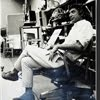## Eugene Ray SDSU 1970'sEugene Ray 1970's. photo credit: Tom Davis

## Thursday, January 10, 2013

### AUDUBON @ OAKLEY plantation DEEP in a west feliciana JUNGLE ! ^^^^^^^^^^^^^^^^^^^^^ OAKLEY in 1821 was IMPRESSIVE OAKLEY in 1954 was POIGNANT ! remote/lost & difficult to locate ^^^^^^^^^^^^^^^^^^^^^ 5 PHOTOS=>AUTUMN OF 1954< #1) oakley stately before a storm #2) the bricks>audubon walked< #3) oakley jungle prior 54 storm #4) oakley jungle after 54 storm #5) radiant light/ post hurricane ^^^^^^^^^^^^^^^^^^^^^ A SURPRISE >AUDUBON PRINT< blue HERON by the river JUNGLE+FELICIANA JUNGLE 1821+
>along the mississippi north of old<
+BATON ROUGE+
>is the setting of the famous<
+OAKLEY PLANTATION+
^^^^^^^^^^^^
>here the talented artist naturalist<
+JEAN JACQUES AUDUBON+
>taught painting to 15 year old<
+ELIZA PIRRIE+
^^^^^^
+HE WAS PAINTING MANY BIRDS+
>feliciana is teeming<
+FOR HIS ELEPHANT FOLIO+
^^^^^^^^^^^^^^
>fast forward<
^^^^^^^
+AUTUMN 1954+
>in my early twenties i was busy<
>photographing plantations<
>w/ a new leica camera<
^^^^^^^^^^^
+DEGRUY=RAY=CAVAROC+
>plantation memories were vital<
>to sunday photo safaris<
^^^^^^^^^^^^^

+AFTER PHOTOGRAPHING FRENCH+
+PLANTATIONS I TURNED TO+
>feliciana jungles<
+ENGLISH PLANTATIONS+
^^^^^^^^^^^^^
>oakley lost deep in a remote<
>feliciana jungle was the<
>most precious find<
^^^^^^^^^^

+TONIGHT'S PHOTOS+
>five photos at oakley before<
>and after the hurricane of<
+AUTUMN 1954+
^^^^^^^^

#1)       +OAKLEY PLANTATION+
(circa 1806)
>before a violent storm<
^^^^^^^^^^^^
>tall and stately, english "tropique"<
>with its louvered sun breaks<
^^^^^^^^^^^^^^^^
>audubon had a small room <
>next to the rear stairs<
^^^^^^^^^^^^

#2)          +ORIGINAL BRICKS+
>walked by audubon and eliza pirrie<
>were still there in the autumn of<
+1954+
>they have since been replaced<
^^^^^^^^^^^^^^^^^

#3)        +MISE-EN-SCENE+
>before the hurricane of autumn<
+1954+
>we drove for miles in my<
+54 PLYMOUTH+
>(french blue w/ white wall tires)<
^^^^^^^^^^^^^^^^^
>it was remote/easy to get lost<
^^^^^^^^^^^^^

#4)         +MISE-EN-SCENE+
>after the hurricane of autumn<
+1954+
>the distruction of the violent<
>storm makes a poignant<
>and poetic inpression<
^^^^^^^^^^^

#5)                +GHOSTLY+
>the jungle still wet after the storm<
>expects audubon & eliza's<
^^^^^^^^^^^^^
+SUDDEN APPEARANCE+
^^^^^^^^^^^^
>alas<
+AUDUBON WAS DISMISSED<
^^^^^^^^^^^^^^^^

>the full story can be read in<
+"A SUMMER OF BIRDS"+
>by danny heitman<
^^^^^^^^^

+POSTSCRIPT+
>i took marianne to oakley and<
>it is completely restored<
(>state park owned<)
^^^^^^^^^^
>WE TOOK THE DELTA QUEEN<
>new orleans/natchez<
^^^^^^^^^^^^^^^

>at vicksburg near rayville we<
+BLUE HERON PRINT+
^^^^^^^^^^
>it arrived at our house upon<
>our return from louisiana<
^^^^^^^^^^^^^
+MARIANNE'S SURPRISE+ !
^^^^^^^^^^^^^

gene ray, lajolla, california

>eugene ray, mfa, architect<
>professor emeritus, sdsu<
^^^^^^^^^^^^^^